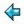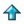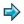### divmodtest.m

```test1 = and [a div b = entier (a/b) | a,b <- [-15..15]; b ~= 0]
test2 = and [b * (a div b) + a mod b = a | a,b <- [-15..15]; b ~= 0]
test3 = and [ ok a b | a,b <- [-15..15]; b ~= 0]
where
ok a b = 0 <= a mod b < b, if b > 0
= b < a mod b <= 0, if b < 0
```

This script defines tests for three properties of div and mod, each checked over a small range of values including various combinations of signs. Each test should yield the result True, or there is something wrong with the arithmetic on your machine!